# Python 将自定义分析推送到 Netezza 数据库

Albury在本篇文章中，你将会了解 ibmdpy4nps 包是什么，以及它是如何帮助机器学习工程师和数据科学家在 Netezza 中执行他们的自定义 ML 和分析功能。Netezza On-Prem 和 Netezza 云版本都支持此功能，提供跨平台的无缝 One Netezza 体验。

## 介绍

### ibmdbpy4nps 与 Netezza 数据库内分析有何不同

Netezza 数据库内分析 (INZA) 是一个极其强大且全面的分析包，它提供了多个足以处理大多数 ML 步骤的 SQL 例程。但是，用户仅限于包中可用的内容。例如，用户可能希望使用他最喜欢的 Python ML 库中可用的最新算法，而不是可用的 INZA 算法来解决 ML 问题。或者他可能想对数据集应用一些自定义转换，这在 INZA 中不可用。这就是 ibmdbpy4nps 包的用武之地。它构建在 Netezza 分析可执行技术之上，允许用户通过简单的 Python 接口直接在数据库内执行自定义 ML 代码。使用 ibmdpy4nps，具有灵活性（用户在数据库内执行的内容方面不受限制），以及速度（你仍然不在客户端运行它，而是在数据库内部运行它）。用户还可以使用 Pandas 数据框样式的抽象连接到数据库表，并将其用于数据探索（已包含多个标准 Pandas 数据框操作的 SQL 转换）。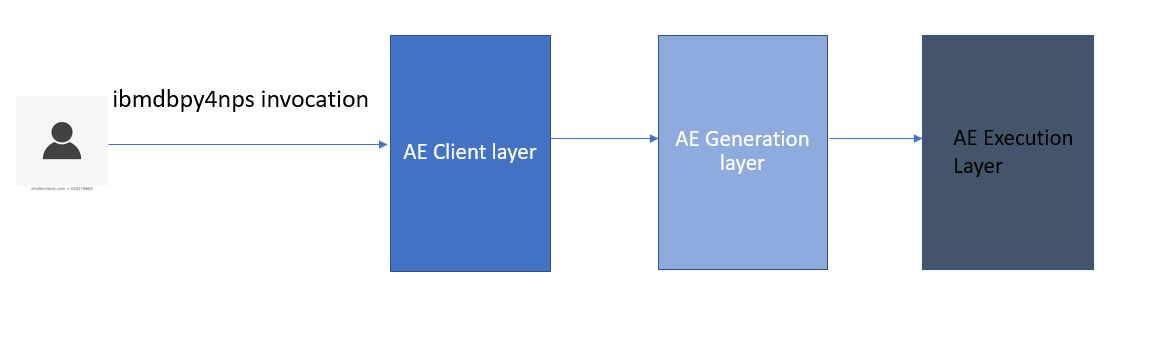• 客户端层——提供用户可以使用所需参数导入和调用的 Python 模块。
• 生成层 — 将客户端的 Python 调用转换为基于分析可执行文件的代码，并添加调用预先存在的用户定义函数所需的 SQL。
• 执行层 — 提供预先存在的用户定义函数，执行 SQL，并运行处理执行的分析可执行启动程序。

### 这个包为数据库内分析提供了什么

1. 允许用户使用 Pandas 风格的数据框抽象连接到数据库表。
2. 支持使用内置 SQL 转换（再次使用 Pandas 数据框样式）的数据库内数据探索。
3. 支持直接在数据库中执行自定义 ML 代码（通过简单的 Python 接口）

## 用例

### 场景

1. 假设你需要对数据库表的每条记录应用自定义数据转换。这不需要在应用例程之前在一个地方聚合数据，因此它可以在不同的数据切片上并行执行。这是一个最佳方案。
2. 假设你需要针对整个数据集构建 ML 模型。这要求在应用模型构建代码之前将数据聚合在一处。这限制了 Netezza 并行运行事物的范围。这不是最佳方案。
3. 假设你的数据可以分区，你的目标是为每个分区构建 ML 模型，每个分区都是一个独立的数据集。在应用模型构建例程之前，这不需要将数据聚合在一处。你可以通过为每个分区并行构建模型来利用 Netezza 并行性。这是一个最佳方案。
4. 假设你需要探索数据，例如收集数据集的统计信息。这要求在应用例程之前将数据聚合在一处，并限制 Netezza 并行运行事物的范围。这不是最佳方案。然而，鉴于这是一项常见任务，我们已经为大多数使用 Pandas 数据帧抽象的数据探索操作提供了数据库内 SQL 实现，用户可以从中受益。

## 安装

1. 在客户端（在 Python 环境中）：使用​`pip install ibmdbpy4nps`​.
2. 在服务器端（Netezza 服务器）：安装从 11.2.1.0 开始的任何 INZA 版本：INZA 自定义（AE 启动器、模板、预注册的 SQL 函数等）在 11.2.1.0 开始的 INZA 版本中可用以支持 ibmdbpy4nps 包调用。
3. 在客户端设置 ODBC 或 JDBC 连接

ODBC 连接：按照安装和配置 ODBC 部分中的步骤设置和配置 ODBC 数据源连接。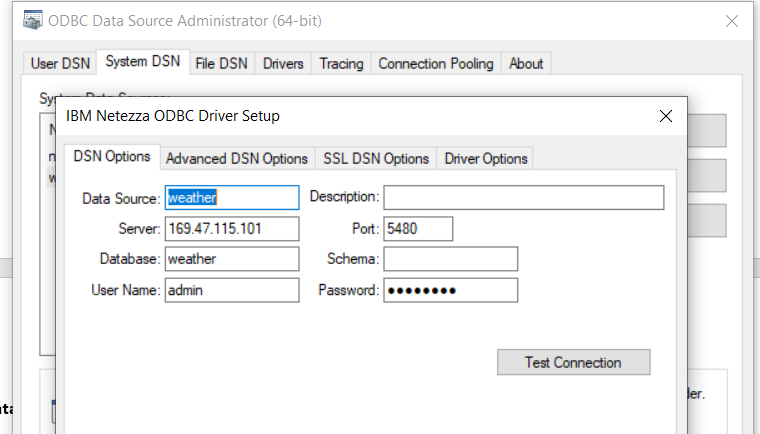JDBC 连接：IBM 知识中心在安装和配置 JDBC部分中描述了如何在你的客户端上安装 Netezza JDBC 驱动程序。下载并安装文件 ​`nzjdbc3.jar`​ 后，你必须将其位置包含在 CLASSPATH 环境变量的值中：

``````export CLASSPATH=<path-to-nzjdbc3.jar>:\$CLASSPATH
``````

``````from ibmdbpy4nps, `import IdaDataBase, IdaDataFrame
``````

``````idadb = IdaDataBase('weather', 'admin', 'password')
``````

``````idadf = IdaDataFrame(idadb, 'WEATHER')
``````

1. 创建一个表：
2. ``create table weather (date Date, Location VARCHAR(100), MinTemp REAL, MaxTemp REAL, Rainfall REAL, Evaporation REAL,Sunshine REAL, WindGustDir VARCHAR(20), WindGustSpeed INTEGER, WindDir9am VARCHAR(10), WindDir3pm VARCHAR(10), WindSpeed9am INTEGER, WindSpeed3pm INTEGER, Humidity9am INTEGER, Humidity3pm INTEGER, Pressure9am REAL, Pressure3pm REAL, cloud9am VARCHAR(10), cloud3pm VARCHAR(10), Temp9am REAL, Temp3pm REAL, RainToday VARCHAR(10), RISK_MM REAL, RainTomorrow VARCHAR(10));``
3. 将 CSV 加载到表中：
4. ``nzload -df weatherAUS.csv -t weather -db weather -pw password -nullValue NA -boolStyle Yes_No -skipRows 1 -delim , -dateStyle MDY -dateDelim '/' ``
5. 对于没有标识列的表（如上面的天气表），创建一个标识列并将值设置为rowid：
6. `` alter table weather add column id bigint; update weather set id=rowid; ``

## 使用内置 SQL 翻译探索数据

`idadf.head()`​— 返回前n行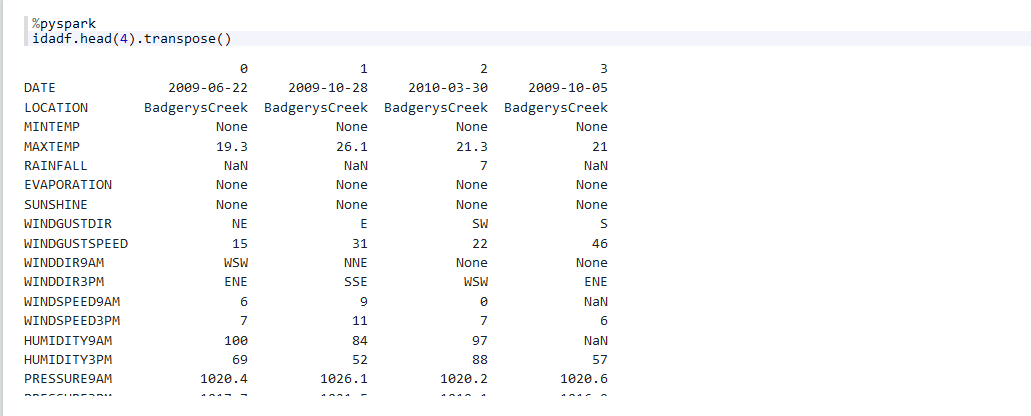`idadf.describe()`​ — 返回列的各种统计信息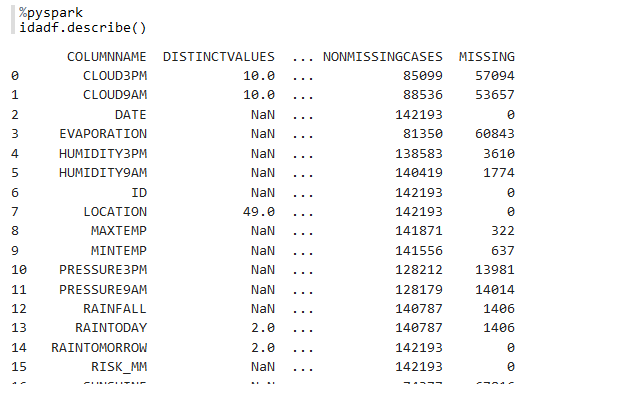`idadf.corr()`​ — 返回列的成对相关性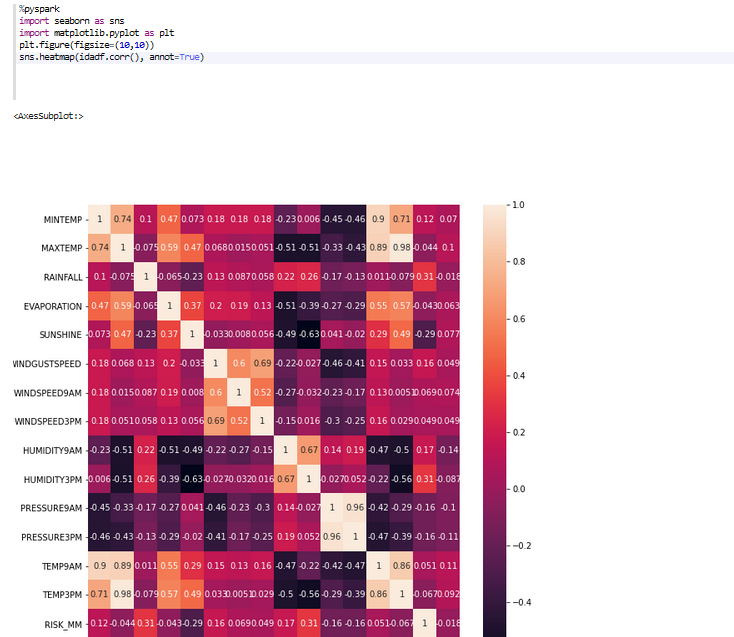## 在数据库内执行自定义分析/机器学习功能

### NZInstall – 在 Netezza 上安装软件包

``````from ibmdbpy4nps.ae.install import NZInstall
# specify the package_name depending on your requirement
package_name=’pandas’
result = nzinstall.getResultCode()``````

### NZFunApply

##### 示例场景：将天气表中的 MAXTEMP 列从摄氏度转换为华氏度

``````from ibmdbpy4nps import IdaDataBase, IdaDataFrame
from ibmdbpy4nps.ae import  NZFunApply

def apply_fun(self, x):
from math import sqrt
max_temp = x
id = x
fahren_max_temp = (max_temp*1.8)+32
row = [id, max_temp,  fahren_max_temp]
self.output(row)

output_signature = {'ID': 'int', 'MAX_TEMP': 'float', 'FAHREN_MAX_TEMP': 'float'}
nz_apply = NZFunApply(df=idadf, fun_ref = code_str_apply, output_table="temp_conversion",output_signature=output_signature, merge_output_with_df=True)
print(result)``````

``````from ibmdbpy4nps import IdaDataBase, IdaDataFrame
from ibmdbpy4nps.ae import  NZFunApply

code_str_apply = """def apply_fun(self, x):
from math import sqrt
max_temp = x
id = x
fahren_max_temp = (max_temp*1.8)+32
row = [id, max_temp,  fahren_max_temp]
self.output(row)
"""
output_signature = {'ID': 'int', 'MAX_TEMP': 'float', 'FAHREN_MAX_TEMP': 'float'}
nz_apply = NZFunApply(df=idadf, code_str=code_str_apply, fun_name='apply_fun', output_table="temp_conversion",output_signature=output_signature, merge_output_with_df=True)
print(result)``````

0 27.700001 81.860001 2008-12-16 0.0 448014
1 33.000000 91.400002 2008-12-22 0.0 448020
2 32.700001 90.860001 2008-12-28 0.0 448026
3 28.799999 83.839996 2009-01-03 0.0 448032
4 28.400000 83.120003 2009-01-09 0.0 448038
142188 32.900002 91.220001 2015-12-02 0.0 589631
142189 37.099998 98.779999 2015-12-08 0.2 589637
142190 39.500000 103.099998 2015-12-14 3.8 是的 589643
142191 30.299999 86.540001 2015-12-20 4.8 是的 589649
142192 36.299999 97.339996 2016-02-06 0.0 589692

### NZFunT申请

#### a) 对数据的每个切片应用该函数

##### 示例场景：转换数据，构建 ML 模型，并测量模型的准确性

``````from ibmdbpy4nps import IdaDataBase, IdaDataFrame
from ibmdbpy4nps.ae import  NZFunTApply

code_str_host_spus="""def decision_tree_ml(self, df):
from sklearn.model_selection import cross_val_score
from sklearn.impute import SimpleImputer
from sklearn.tree import DecisionTreeClassifier

from sklearn.preprocessing import LabelEncoder
import numpy as np

location = df.LOCATION

# data preparation
imputed_df = df.copy()
ds_size = len(imputed_df)
imputed_df['CLOUD9AM'] = imputed_df.CLOUD9AM.astype('str')
imputed_df['CLOUD3PM'] = imputed_df.CLOUD3PM.astype('str')
imputed_df['SUNSHINE'] = imputed_df.SUNSHINE.astype('float')
imputed_df['EVAPORATION'] = imputed_df.EVAPORATION.astype('float')

#remove columns which have only null values
columns = imputed_df.columns
for column in columns:
if imputed_df[column].isnull().sum()==len(imputed_df):
imputed_df=imputed_df.drop(column, 1)

columns = imputed_df.columns

for column in columns:

if (imputed_df[column].dtype == 'float64' or imputed_df[column].dtype == 'int64'):
imp = SimpleImputer(missing_values=np.nan, strategy='mean')
imputed_df[column] = imp.fit_transform(imputed_df[column].values.reshape(-1, 1))

if (imputed_df[column].dtype == 'object'):
# impute missing values for categorical variables
imp = SimpleImputer(missing_values=None, strategy='constant', fill_value='missing')
imputed_df[column] = imp.fit_transform(imputed_df[column].values.reshape(-1, 1))
imputed_df[column] = imputed_df[column].astype('str')
le = LabelEncoder()
#print(imputed_df[column].unique())

le.fit(imputed_df[column].unique())
# print(le.classes_)
imputed_df[column] = le.transform(imputed_df[column])

X = imputed_df.drop(['RISK_MM', 'RAINTOMORROW'], axis=1)
y = imputed_df['RAINTOMORROW']

# Create a decision tree
dt = DecisionTreeClassifier(max_depth=5)

cvscores_3 = cross_val_score(dt, X, y, cv=3)

self.output(ds_size, location, np.mean(cvscores_3))
"""``````

``````output_signature = {'DATASET_SIZE': 'int', 'LOCATION':'str', 'CLASSIFIER_ACCURACY':'double'}

nz_fun_tapply = NZFunTApply(df=idadf, code_str=code_str_host_spus, fun_name ="decision_tree_ml", parallel=True, output_signature=output_signature)
result = nz_fun_tapply.get_result()
print(result)``````

0 23673 Albury 0.824822
1 23734 Albury
0.827126
2 23686 Albury
0.813898
3 23706 Albury
0.818485
4 23739 Albury
0.832175
5 23655 Albury
0.826168

#### b) 应用于数据集的函数

``````output_signature = {'DATASET_SIZE': 'int', 'LOCATION':'str', 'CLASSIFIER_ACCURACY':'double'}
nz_fun_tapply = NZFunTApply(df=idadf, code_str=code_str_host_spus, fun_name ="decision_tree_ml", parallel=False, output_signature=output_signature)
result = nz_fun_tapply.get_result()
print(result)``````

142193 Albury
0.827115

### NZFunGroupedApply

• `NZFunTApply `​使用静态切片，这意味着这些切片可能不是你的场景所需的数据排列。
• 该函数是针对整个切片执行的，而不是针对切片中的组执行的。
##### 示例场景：转换数据、构建 ML 模型并为模型评分

``````from ibmdbpy4nps import IdaDataBase, IdaDataFrame
from ibmdbpy4nps.ae import  NZFunGroupedApply

code_str_host_spus="""def decision_tree_ml(self, df):
from sklearn.model_selection import cross_val_score
from sklearn.impute import SimpleImputer
from sklearn.tree import DecisionTreeClassifier
from sklearn.model_selection import train_test_split

from sklearn.preprocessing import LabelEncoder
import numpy as np

# data preparation
imputed_df = df.copy()
ds_size = len(imputed_df)
temp_dict = dict()

columns = imputed_df.columns

for column in columns:
if column=='ID':
continue

if (imputed_df[column].dtype == 'float64' or imputed_df[column].dtype == 'int64'):
if imputed_df[column].isnull().sum()==len(imputed_df):
imputed_df[column] = imputed_df[column].fillna(0)

else :

imp = SimpleImputer(missing_values=np.nan, strategy='mean')
transformed_column = imp.fit_transform(imputed_df[column].values.reshape(-1, 1))
imputed_df[column] = transformed_column

if (imputed_df[column].dtype == 'object'):
# impute missing values for categorical variables
imp = SimpleImputer(missing_values=None, strategy='constant', fill_value='missing')
imputed_df[column] = imp.fit_transform(imputed_df[column].values.reshape(-1, 1))
imputed_df[column] = imputed_df[column].astype('str')
le = LabelEncoder()

le.fit(imputed_df[column])
# print(le.classes_)
imputed_df[column] = le.transform(imputed_df[column])
temp_dict[column] = le

# Create a decision tree
dt = DecisionTreeClassifier(max_depth=5)
X = imputed_df.drop(['RISK_MM', 'RAINTOMORROW'], axis=1)
y = imputed_df['RAINTOMORROW']
X_train, X_test, y_train, y_test = train_test_split(X,y, test_size = 0.25, random_state=42, stratify=y)

dt.fit(X_train, y_train)

accuracy = dt.score(X_test, y_test)

pred_df = X_test.copy()

y_pred= dt.predict(X_test)

pred_df['RAINTOMORROW'] = y_pred
pred_df['DATASET_SIZE'] = ds_size
pred_df['CLASSIFIER_ACCURACY']=round(accuracy,2)

original_columns = pred_df.columns

for column in original_columns:

if column in temp_dict:
pred_df[column] = temp_dict[column].inverse_transform(pred_df[column])
#print(pred_df)

def print_output(x):
row = [x['ID'], x['RAINTOMORROW'], x['DATASET_SIZE'], x['CLASSIFIER_ACCURACY']]
self.output(row)

pred_df.apply(print_output, axis=1)

"""

output_signature = {'ID':'int', 'RAINTOMORROW_PRED' :'str',  'DATASET_SIZE':'int', 'CLASSIFIER_ACCURACY':'float'}

nz_groupapply = NZFunGroupedApply(df=idadf,  code_str=code_str_host_spus, index='LOCATION', fun_name="decision_tree_ml", output_signature=output_signature, merge_output_with_df=True)

print(result)``````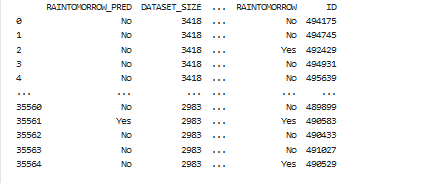### Netezza安装

``````from ibmdbpy4nps.ae.install import NZInstall